论文阅读 - Panoptic Segmentation 全景分割

Panoptic Segmentation 全景分割

• 统一了实例分割(Instance Segmentation) 和语义分割(Semantic Segmentation).
• 实例分割 - 检测每个 object instance，并进行分割；
• 语义分割 - 对每个像素分类.

• basic 算法 —— 结合实例分割和语义分割的全景分割方法，输出全景结果.

• CV 以往主要关注于 things - 可数的 objects (countable obects)，如 people, animals, tools 等.
• 而对于 stuff - 相同或相似纹理或材料的不规则区域，如 grass，sky，road 等的关注较少.
• 关于 Stuff 的研究主要是以语义分割的进行，如 Figure 1b. 其目的是，通过对图片中每一个像素分类，来确定不规则、无组织、不可数的 stuff；语义分割方法是将 thing categories 作为 stuff.
• 关于 Things 的研究可以作为目标检测或实例分割进行，如Figure 1c. 其目的是，通过检测每个 object，并以 bounding box 或 segmentation mask 的方式描述.

• Can there be a reconciliation between stuff and things?
• Is there a simple problem formulation that gracefully encompasses both tasks?
• And what would a unified visual recognition system look like?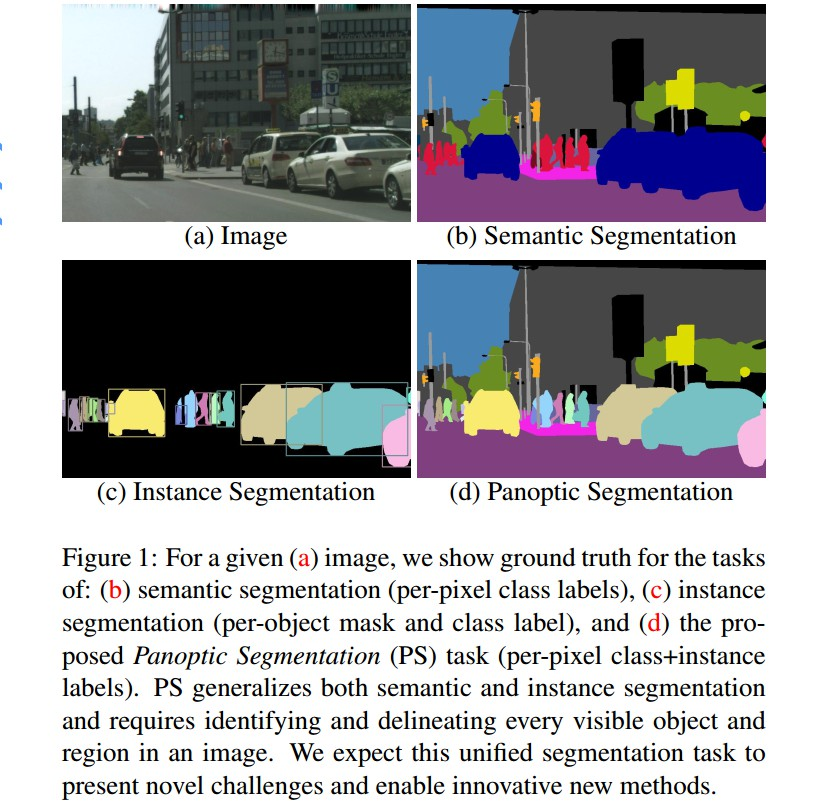Figure1. (a) 给定图片；(b)语义分割 groundtruth，逐像素的 class labels；(c)实例分割 groundtruth，逐 object 的 mask 和 class label；(d) 全景分割 groundtruth，逐像素的 class labels 和 instance labels. PS 将语义分割和实例分割泛化，对图片中的每一个可见 object 和 region 进行辨别与描述.

• 对比语义分割，全景分割需要区分不同的 object instances；对于 FCN-based 方法具有挑战性.
• 对比实例分割，全景分割必须是非重叠的(non-overlapping)；对于 region-based 方法具有挑战性.
• 全景分割需要同时识别 stuff 和 things.

1. 全景分割

1.2 Stuff 和 Thing Labels

${ \mathcal{L}^{St} }$ 和 ${ \mathcal{L}^{Th} }$ 分别表示 stuff labels 和 thing labels.

1.3 与语义分割区别

• 均需要对图像的每个像素设定 semantic label.
• 如果 groundtruth 未指定 instances 信息，或者所有的 categories 都是 stuff，二者相同.

• 当有 thing categories 时，图片中有多个 instances 时，则二者有区别.

1.4 与实例分割区别

• 实例分割 - 对图片中的每个 object 进行分割，允许 objects 重叠(overlapping)；
• 全景分割 - 图片每个像素只有一个 semantic label 和 一个 instance id. 不允许重叠.

2. Panoptioc Quality

 - Completeness

 - Interpretability

 - Simplicity

PQ 计算预测的全景分割与 groundtruth 的差异. 主要包括两步：

 - instance matching 实例匹配
 - 给定 mathes，计算PQ.

2.1 Instance Matching

Theorem1.

${ IoU(p_i, g) = \frac{|p_i \cap g|}{|p_i \cup g|} \le \frac{|p_i \cap g|}{|g|}, i \in \lbrace 1, 2\rbrace }$

${ IoU(p1, g) + IoU(p2, g) \le \frac{|p1 \cap g| + |p2 \cap g|}{|g|} \le 1 }$

Theorem 1 给出了全景分割度量评价需要的两种特点：

• 简单有效 - segment 是唯一且易于计算的.
• 可解释且易于理解

2.2 PQ 计算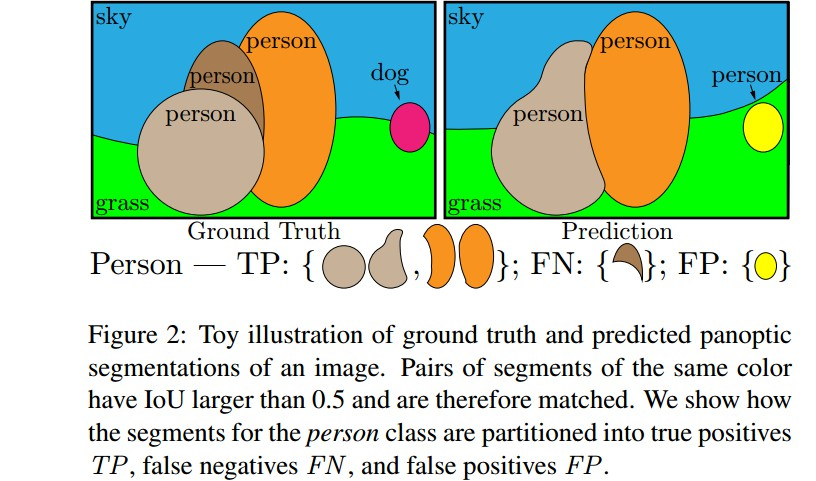Figure 2. 图片全景分割的 groundtruth 和 predicted 结果. 相同颜色的 segments pairs 的 IoU 大于 0.5，被匹配到. 这里给出了 person 类的 segments 划分为 TP、FN 和 FP 的例子.

${ PQ = \frac{\sum_{(p,g) \in TP} IoU(p, g)}{|TP| + \frac{1}{2} |FP| + \frac{1}{2} |FN|} }$

${ \frac{1}{|TP|} \sum_{(p,g) \in TP} IoU(p, g) }$ 是匹配 segments 的平均 IoU；

${ \frac{1}{2} |FP| + \frac{1}{2} |FN| }$ 是惩罚(penalize) 没有匹配的 instances.

PQ 计算的等价形式：

${ PQ = \frac{\sum_{(p,g) \in TP} IoU(p, g)}{|TP| } × \frac{|TP|}{|TP|+ \frac{1}{2} |FP| + \frac{1}{2} |FN|} }$

${ SQ = \frac{\sum_{(p,g) \in TP} IoU(p, g)} {|TP| } }$

${ DQ = \frac{|TP|}{|TP|+ \frac{1}{2} |FP| + \frac{1}{2} |FN|} }$

SQ 是匹配 objects 的平均 IoU.

DQ 类似于 F1-score，在检测任务中被用到.

${ PQ = SQ × DQ }$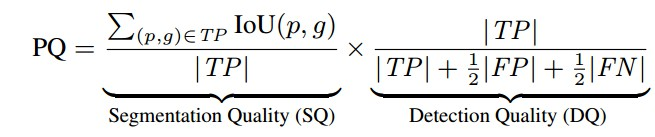3. 全景分割数据集

 - Cityscapes
5000 张图片，2975 张 train，500 张 validation， 1525 张 test.

25k 张图片，20k 张 train， 2k val，3k test.

 - Mapillary Vistas
25k 张街景图片， 18k 张 train，2k 张 val，5k 张 test.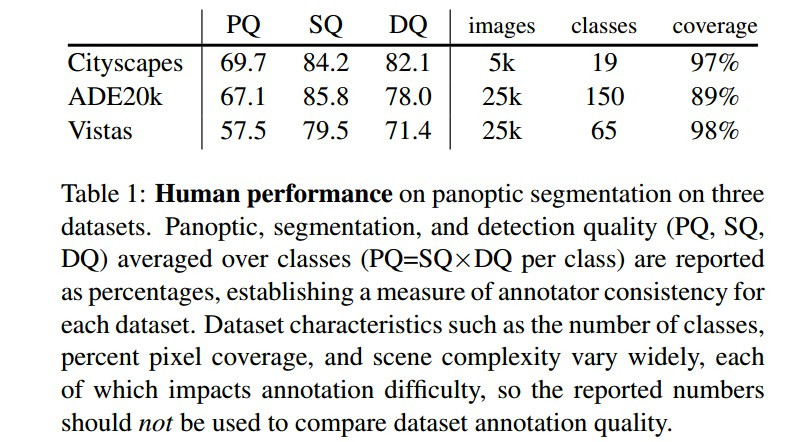4. Results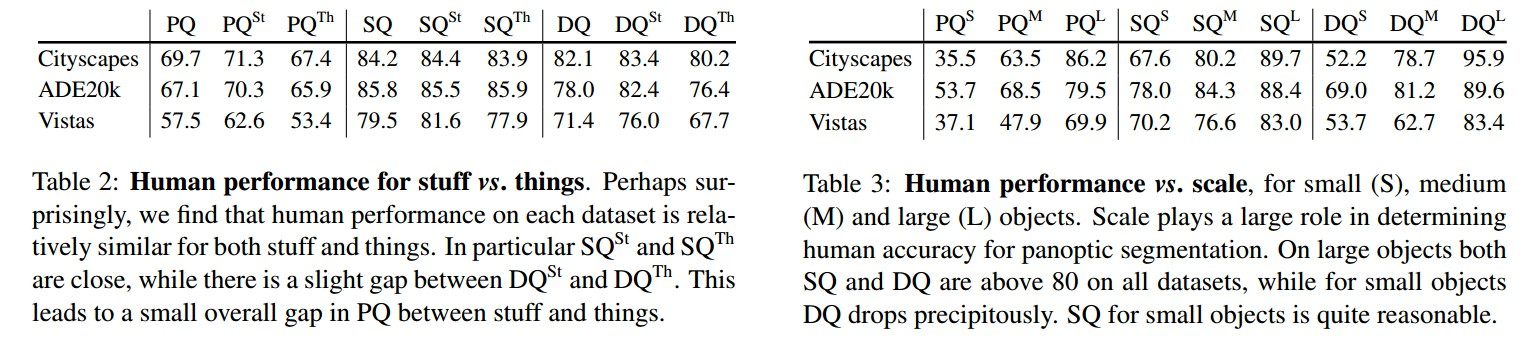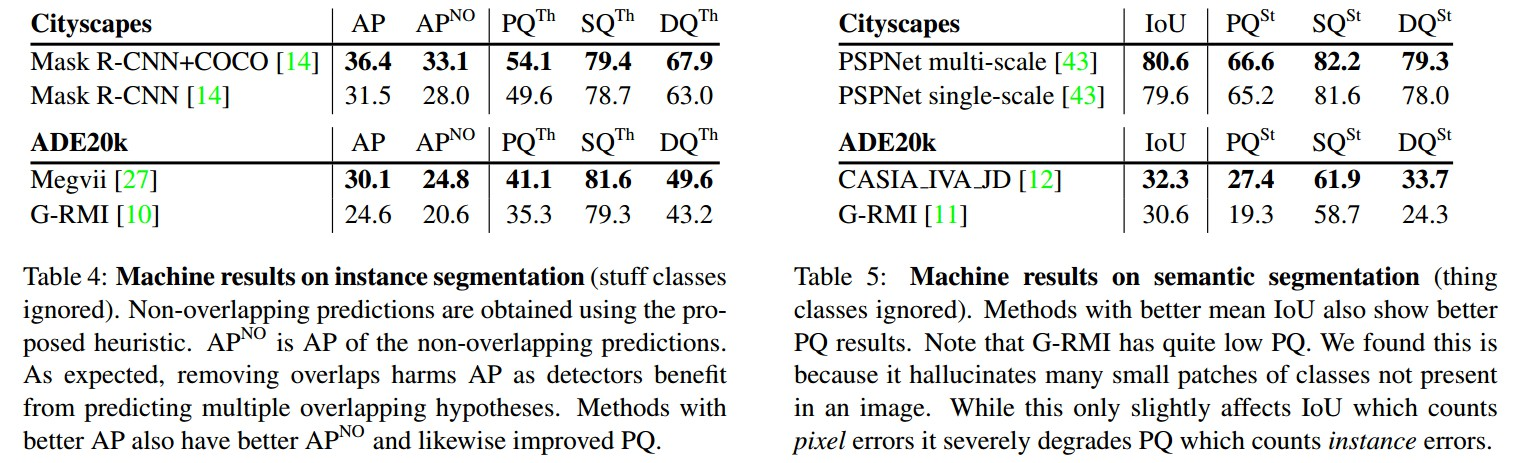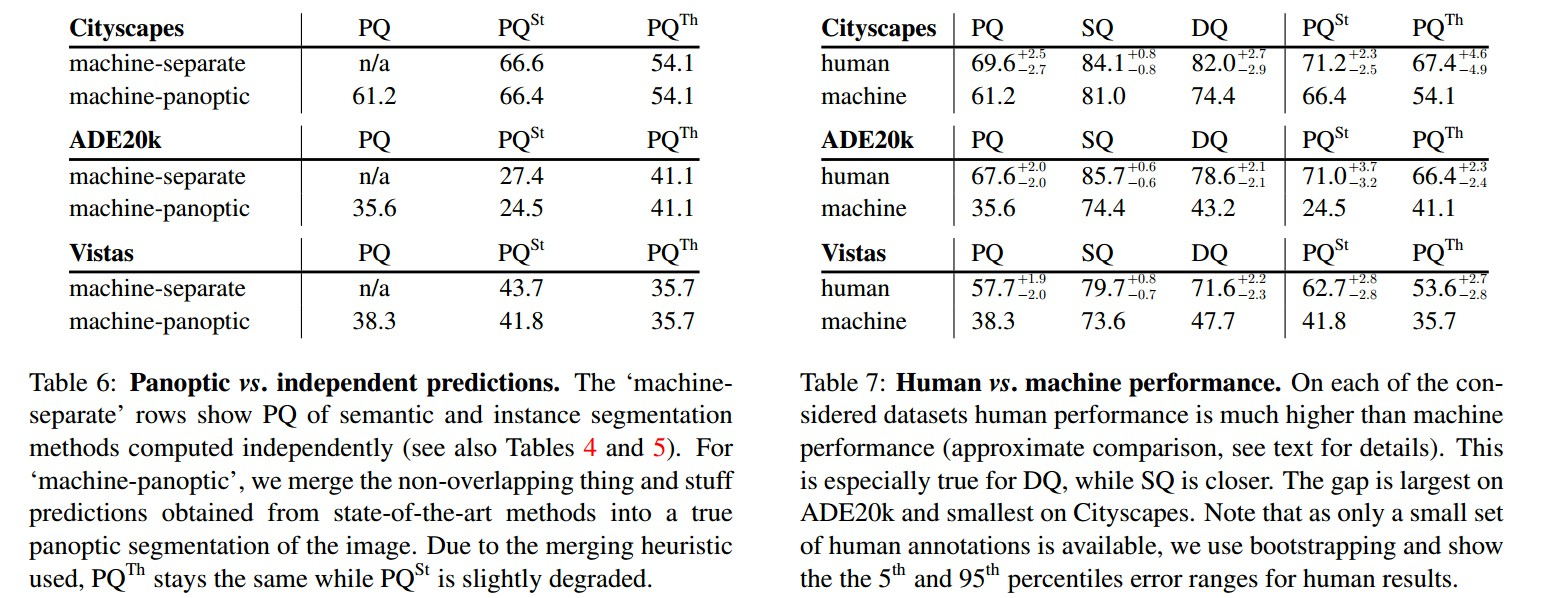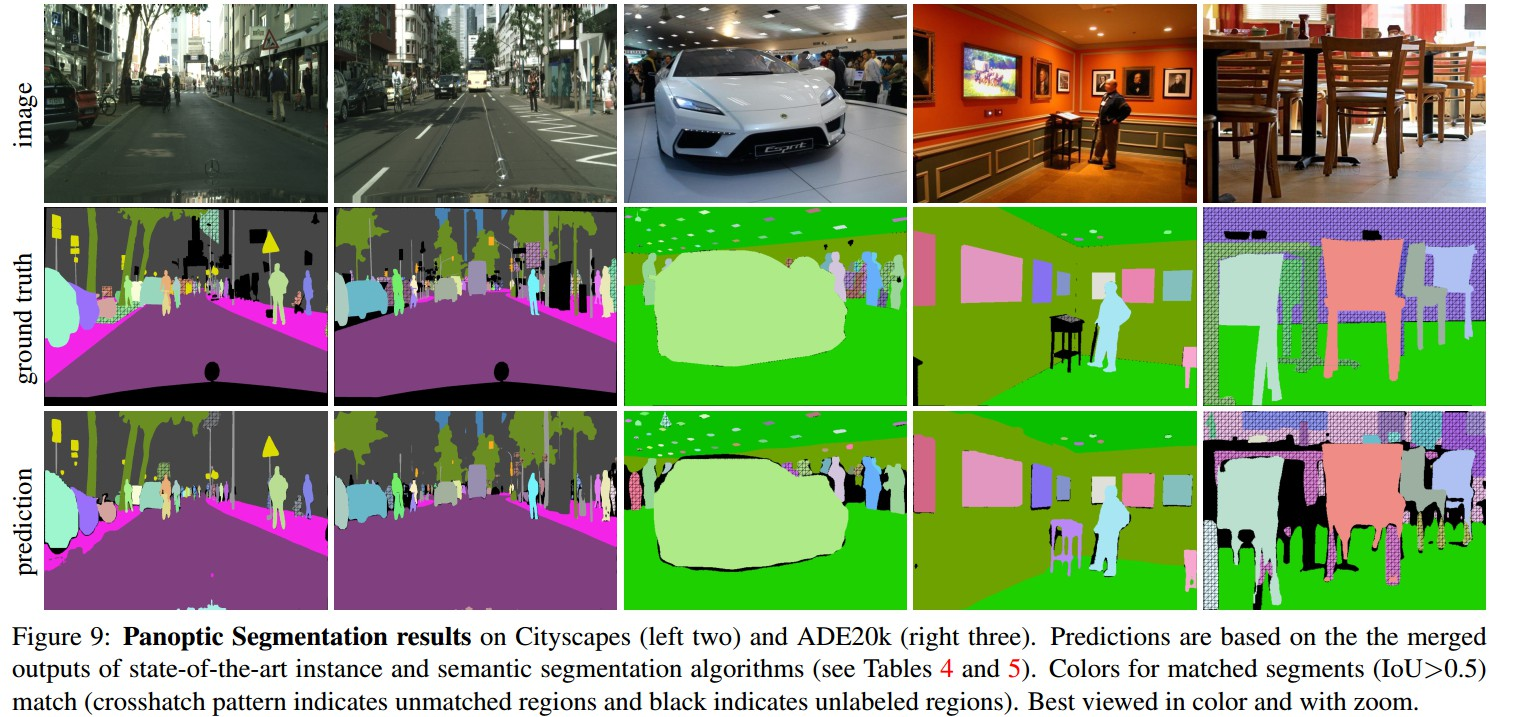5. 全景分割的前景

PS baseline 算法仅是结合实例分割和语义分割的输出，其创新方向可有：

• 深度 End-to-end 模型，以同时处理 PS 中的 stuff-and-thing；
• 由于PS 不能有重叠 segments，高层的推理可能有帮助解决. 如 learnable NMS.
Last modification：October 10th, 2018 at 01:24 am